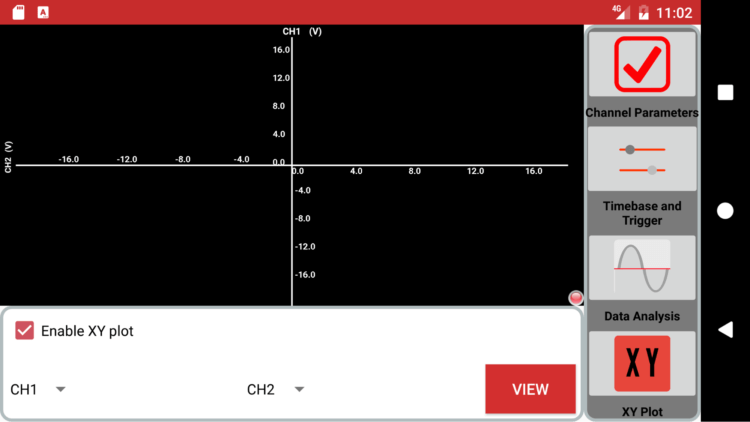# Creating a Four Quadrant Graph for PSLab Android App

While working on Oscilloscope in PSLab Android, we had to implement XY mode. XY plotting is part of regular Oscilloscope and in XY plotting the 2 signals are plotted against each other. For XY plotting we require a graph with all 4 quadrants but none of the Graph-View libraries in Android support a 4 quadrants graph. We need to find a solution for this. So, we used canvas class to draw a 4 quadrants graph.  The Canvas class defines methods for drawing text, lines, bitmaps, and many other graphics primitives. Let’s discuss how a 4 quadrants graph is implemented using Canvas.

Initially, a class Plot2D extending View is created along with a constructor in which context, values for X-Axis, Y-Axis.

```public class Plot2D extends View {
public Plot2D(Context context, float[] xValues, float[] yValues, int axis) {
super(context);
this.xValues = xValues;
this.yValues = yValues;
this.axis = axis;
vectorLength = xValues.length;
paint = new Paint();
getAxis(xValues, yValues);
}```

So, now we need to convert a particular float value in a pixel. This is the most important part and for this, we create a function where we send the value of the pixels, the minimum and the maximum value of the axis and array of float values. We get an array of converted pixel values in return. p[i] = .1 * pixels + ((value[i] – min) / (max – min)) * .8 * pixels; is the way to transform an int value to a respective pixel value.

```private int[] toPixel(float pixels, float min, float max, float[] value) {
double[] p = new double[value.length];
int[] pInt = new int[value.length];

for (int i = 0; i < value.length; i++) {
p[i] = .1 * pixels + ((value[i] - min) / (max - min)) * .8 * pixels;
pInt[i] = (int) p[i];
}
return (pInt);
}
```

For constructing a graph we require to create the axis, add markings/labels and plot data in the graph. To achieve this we will override onDraw method. The parameter to onDraw() is a Canvas object that the view can use to draw itself. First, we need to get various parameters like data to plot, canvas height and width, the location of the x axis and y axis etc.

```@Override
protected void onDraw(Canvas canvas) {

float canvasHeight = getHeight();
float canvasWidth = getWidth();
int[] xValuesInPixels = toPixel(canvasWidth, minX, maxX, xValues);
int[] yValuesInPixels = toPixel(canvasHeight, minY, maxY, yValues);
int locxAxisInPixels = toPixelInt(canvasHeight, minY, maxY, locxAxis);
int locyAxisInPixels = toPixelInt(canvasWidth, minX, maxX, locyAxis);```

Drawing the axis

First, we will draw the axis and for this, we will use the white color. To draw the white color axis line we will the following code.

```paint.setColor(Color.WHITE);
paint.setStrokeWidth(5f);
canvas.drawLine(0, canvasHeight - locxAxisInPixels, canvasWidth,
canvasHeight - locxAxisInPixels, paint);
canvas.drawLine(locyAxisInPixels, 0, locyAxisInPixels, canvasHeight,
paint);```

After drawing the axis lines, now we need to mark labels for both x and y axis. For this, we use the following code in onDraw method. By this, the axis labels are automatically marked after a fixed distance. The no. of labels depends on the value of n. The code ensures that the markings are apt for each of the quadrant, for example in the first quadrant the markings of the x axis is below the axis, whereas markings of the y axis are to the left.

```float temp = 0.0f;
int n = 8;
for (int i = 1; i <= n; i++) {
if (i <= n / 2) {
temp = Math.round(10 * (minX + (i - 1) * (maxX - minX) / n)) / 10;
canvas.drawText("" + temp,
(float) toPixelInt(canvasWidth, minX, maxX, temp),
canvasHeight - locxAxisInPixels - 10, paint);
temp = Math.round(10 * (minY + (i - 1) * (maxY - minY) / n)) / 10;
canvas.drawText("" + temp, locyAxisInPixels + 10, canvasHeight
- (float) toPixelInt(canvasHeight, minY, maxY, temp),
paint);
} else {
temp = Math.round(10 * (minX + (i - 1) * (maxX - minX) / n)) / 10;
canvas.drawText("" + temp,
(float) toPixelInt(canvasWidth, minX, maxX, temp),
canvasHeight - locxAxisInPixels + 30, paint);
temp = Math.round(10 * (minY + (i - 1) * (maxY - minY) / n)) / 10;
canvas.drawText("" + temp, locyAxisInPixels - 65, canvasHeight
- (float) toPixelInt(canvasHeight, minY, maxY, temp),
paint);```

By using this code we get the following resultsPlotting the data

The last step is to plot the data, to achieve this we first convert float values of x axis and y axis data point to pixels using toPixel method and simply draw it on the graph. In addition to this, we set a red color to the line.

```paint.setStrokeWidth(2);
canvas.drawARGB(255, 0, 0, 0);
for (int i = 0; i < vectorLength - 1; i++) {
paint.setColor(Color.RED);
canvas.drawLine(xValuesInPixels[i], canvasHeight
- yValuesInPixels[i], xValuesInPixels[i + 1], canvasHeight
- yValuesInPixels[i + 1], paint);
}```

This implements a 4 quadrants graph in PSLab Android app for XY plotting in Oscilloscope Activity. The entire code for the same is available in here.